[email protected] +1 203-349-9909 / +91-8080042523

# Selecting Multiple Options in Dependent Drop Down List Box using VBA

In our earlier blog we have already discussed how to create a dependent drop down list box in Excel and as well as in VBA when we are using the list box in VBA.

Now in this blog, we will discuss how to select multiple options from the dependent drop down list Box as it helps to send out the customized message or perform an action based on multiple selections made by users.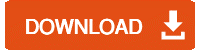Hope you have the same file which we have used earlier.

## Now let’s start steps by step to create a Dependent drop down list box:

We are creating a Dependent list box with multiple options (items). From the below user form we will select the 2 values (items), to get a custom message.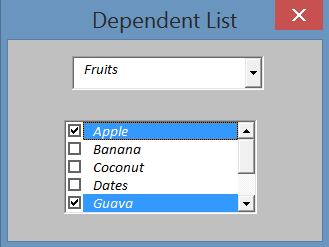(Picture 1)

It is very easy to select the value, but to use the same value to send a message or call for an action is not very easy. For example, We want to show the value in the message box like “I like Apple and Guava

To do the same we are using a command button (OK) in the form. Now our form looks like this: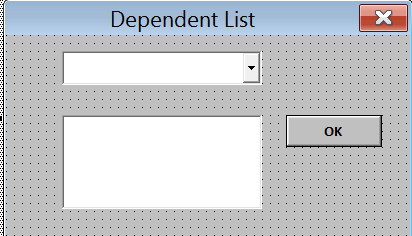(Picture 2)

So if you write the below code like we use for selecting the value it will not work.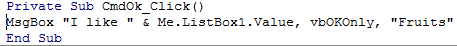(Picture 3)

Now we have written the code and try to run the code. But we got only “I like” in the message box (It’s a problem).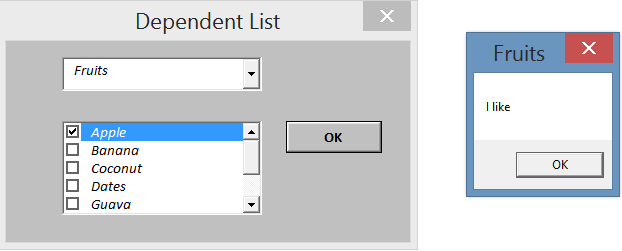(Picture 4)

### So now we have to change the code to get the selected value in the message box by these steps:

1. Double Click on “OK” button.
2. Paste the below code
3. Run the Form
4. Select values from the dependent drop down list
5. Then Press OK button

#### Here is the code:

```Private Sub CmdOk_Click()
Dim First As Boolean
Dim i As Long
Dim List As String
First = True
With ListBox1
For i = 0 To .ListCount - 1
If .Selected(i) Then
If First Then
List = .List(i)
First = False
Else
List = List & "," & .List(i)
End If
End If
Next i
End With
MsgBox "I like " & List, vbOKOnly, "Fruits"
End Sub

```

Code will like below image: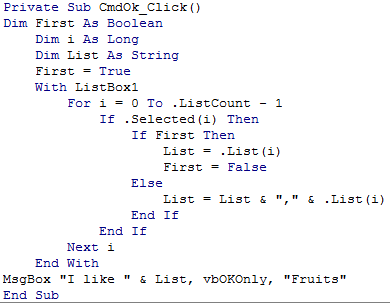(Picture 5)

### Related blog on: How to split Excel data into separate sheets using VBA?

Now let’s run the code and then select the values from the dependent list and then press “OK” button.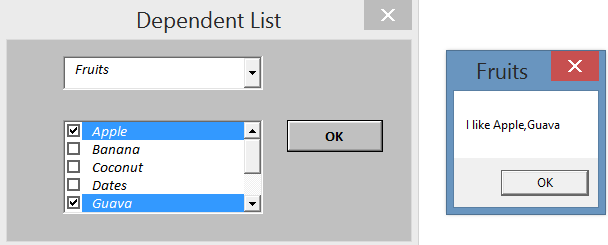(Picture 6)

As we have selected “Apple” and “Guava” and we are getting the same value in the msg box. Now If we choose any values from the dependent list and then press the “OK” button, then it will only show the value which we will select.

We found one bug (problem) in this code. The bug is that if we don’t choose any value from the dependent list and press “OK” button then it will show the below message.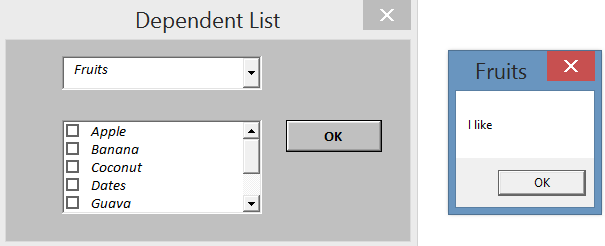(Picture 7)

So we need to apply another data validation for multiple selections over here so that if we don’t select any value from the dependent list box because it will give us the message that we must select a value from the dependent list. We need to change the code.

Here is the revised code:

```Private Sub CmdOk_Click()
Dim First As Boolean
Dim i As Long
Dim List As String
First = True
With ListBox1
For i = 0 To .ListCount - 1
If .Selected(i) Then
If First Then
List = .List(i)
First = False
Else
List = List & "," & .List(i)
End If
End If
Next i
End With
If List = "" Then
MsgBox "You must select an item!!!", vbExclamation, "Item"
Exit Sub
End If
MsgBox "I like " & List, vbOKOnly, "Fruits"
End Sub

```

### This time, our code looks like this: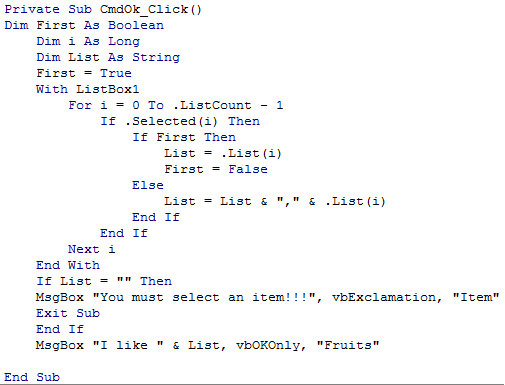(Picture 8)

Now if we don’t select any value then, this time, we will get a message that we need to select at least one value from the list box: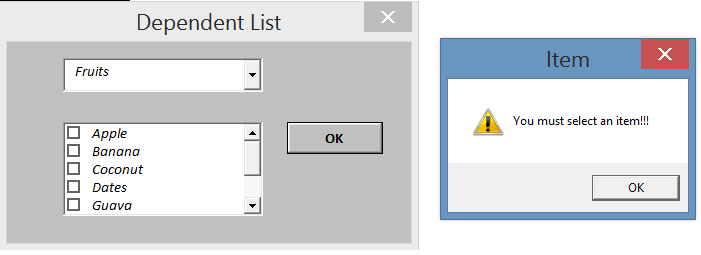(Picture 9)

We can also use another value also like this way. Just give the same name for all the boxes we have used in the form and then use the same code in the list box.

Finally, we can guarantee that you will enjoy preparing such kind of reports using VBA with our advanced Excel VBA tutorials.

You can also check out our: FREE Excel VBA Tutorial